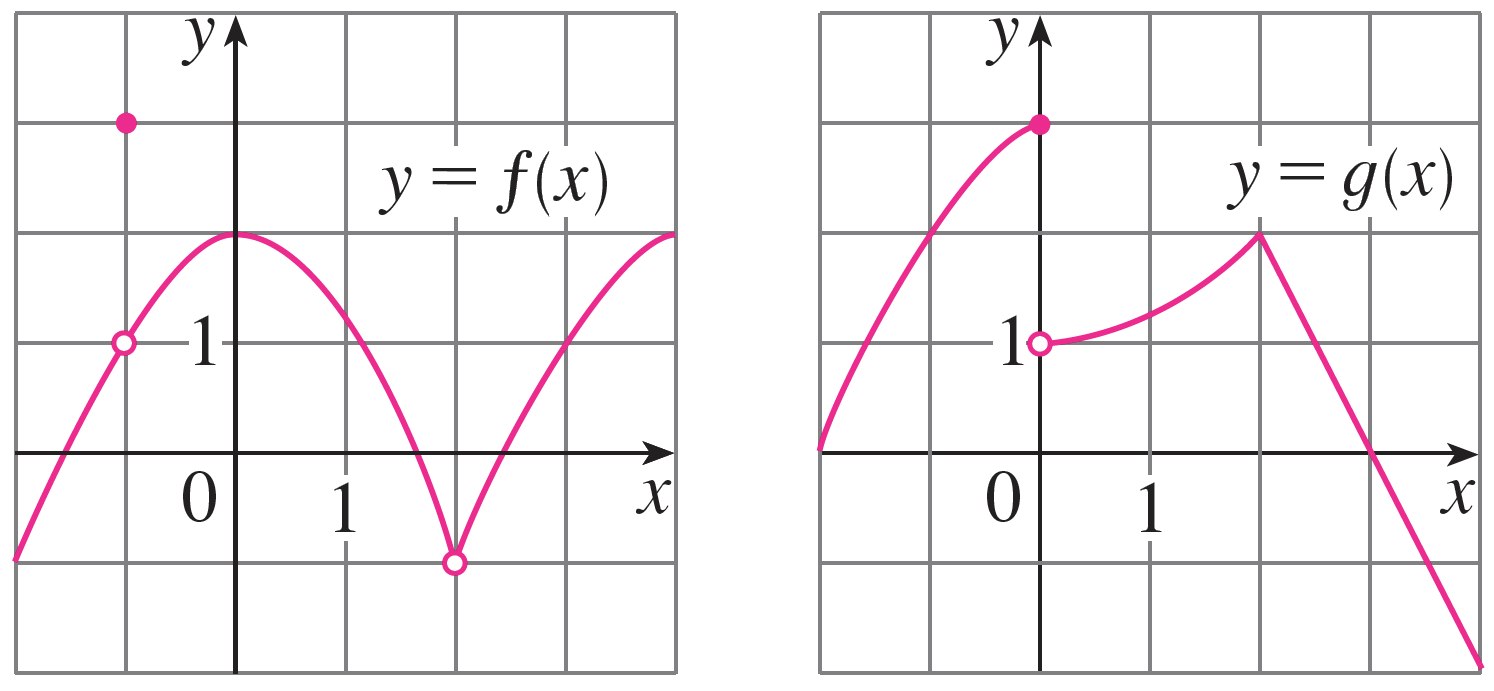# Homework 2

Directions: Show each step of your work. Turn in your answers in class on a physical piece of paper. Staple multiple sheets together. Feel free to use Desmos for graphing.

1. Suppose $f(x) = x^2 + 2 \qquad g(x) = \dfrac{x + 1}{x - 1}$
1. Find $f+g$, $f/g$ and their domains.
2. Find $f\circ g, g\circ f, (f\circ g)(5), (g\circ f)(5)$.
2. For the next few parts, find $\dfrac{f(a + h) - f(a)}{h},\qquad h \neq 0$ and simplify fully:
1. $f(x) = x^2$
2. $f(x) = \dfrac{1}{x}$
3. $f(x) = x^3 - 1$
3. For the next few parts, find two functions $f$ and $g$ such that $h = f\circ g$. You are not allowed to choose $g(x) = x$.
1. $h(x) = (x^3 + x + 1)^3$
2. $h(x) = \dfrac{1}{\sqrt{x^2 - 2}}$
3. $h(x) = \dfrac{1}{\sqrt{x + 1}} + \sqrt{x + 1}$
4. Apple manufactures smartphones at a variable cost of $V(x) = 0.000003x^3 - 0.03x^2 + 200x$ dollars and has a monthly fixed cost of $\$90,000$. The total revenue function $R(x) = -0.1x^2 + 500x, \qquad 0 \leq x \leq 5000$ 1. Find a function$C$that gives the total cost incurred by manufacturing$x$smartphones. 2. What is the total cost when 2000 smartphones are manufactured? 3. Find the total profit function and its domain. 4. What is the total profit when 2000 smartphones are manufactured and sold each month? 5. The revenue of Expedia is given by$f(x)$, where$x$is the total USD spent on advertising. The function$g(t)$is the amount Expedia spends on advertising at time$t$. What does$f\circ g$mean in English? 6. A hotel in San Luis Obispo has an estimated occupancy rate given by the function $o(t) = \dfrac{1}{8}t^3-3t^2+20t + 55, \qquad 0 \leq t \leq 11$ where$t$is measured in months and$t = 0$is January. Based on historic data, hotel management estimates the monthly revenue is approximated by the function $R(x) = -\frac{1}{1000}x^{3}+\frac{1}{5}x^{2}, \qquad 0 \leq x \leq 100$ where$x$(in percent) is the occupancy rate. 1. What is the hotel's occupancy rate in January? How about in June? 2. Graph$o(t)$on the coordinate plane. In what month does the maximum occupancy occur? 3. What is the hotel's monthly revenue in January and in June? 7. Say whether the following statements are true or false. If they are false, give one counterexample (example that shows the statement has to be false). 1. If$f$is a function, then it is always true that$f \circ f = f\cdot f$. 2. If$f$and$g$are functions, then it is always true that$f\circ g = g\circ f$. 8. Find the following limits using the table method. If they do not exist, explain why. 1.$\lim_{x \rightarrow 2} (x^2 + 1)$2.$\lim_{x \rightarrow 2} \dfrac{1}{x-2}$3.$\lim_{x \rightarrow 0} \dfrac{\lvert x \rvert}{x}$9. Given these functionsfind the limits. If they do not exist, explain why they do not. 1.$\displaystyle\lim_{x \rightarrow 2}[f(x) + g(x)]$2.$\displaystyle\lim_{x \rightarrow -1}[f(x)g(x)]$3.$\displaystyle\lim_{x \rightarrow 3}[f(-1) + g(x)]$4.$\displaystyle\lim_{x\rightarrow 2}[x^2 \cdot f(x)]$5.$\displaystyle\lim_{x\rightarrow 0}[f(x) + g(x)]$6.$\displaystyle\lim_{x\rightarrow 3}\left[\dfrac{f(x)}{g(x)}\right]$10. Find the following limits using the limit laws and fully simplify. State which laws you are using (refer to Lecture 2.4) for each equal sign. 1.$\displaystyle\lim_{x\rightarrow 1} (x + 1)$2.$\displaystyle\lim_{t\rightarrow 0} (4t^2 + 2t + 1)$3.$\displaystyle\lim_{x\rightarrow 1} (x + 1)$4.$\displaystyle\lim_{x\rightarrow 3} (2x + 1)(x-1)(x+2)^2$5.$\displaystyle\lim_{x\rightarrow 2} \sqrt{x + 2}$6.$\displaystyle\lim_{x\rightarrow 1} \sqrt{\dfrac{2x^2 + 2}{2x^2 - 1}}$7.$\displaystyle\lim_{x\rightarrow -1}\dfrac{x^2 - 1}{x + 1}$8.$\displaystyle\lim_{x\rightarrow 0}\dfrac{2x^2 + x}{x}$9.$\displaystyle\lim_{x\rightarrow -3}\dfrac{x+1}{x-3}$11. For the following problems, sketch the graph of$f$and find$\displaystyle \lim_{x\rightarrow a} f(x)$, if it exists. 1.$f(x) = \begin{cases}x + 1 & x \leq 0 \\ 0 & x > 0\end{cases}, \qquad a = 0$2.$f(x) = \begin{cases}\lvert x \rvert & x \leq 2 \\ 2 & x > 2\end{cases}, \qquad a = 2$3.$f(x) = \begin{cases} x^2 & x \leq 1 \\ x-1 & x > 1\end{cases}, \qquad a = 1$4.$f(x) = \begin{cases} x^2 - 3 & x \neq 0 \\ 0 & x = 0\end{cases}, \qquad a = 0\$